Next: The conical pendulum Up: Circular motion Previous: Uniform circular motion

## Centripetal acceleration

An object executing a circular orbit of radiuswith uniform tangential speedpossesses a velocity vectorwhose magnitude is constant, but whose direction is continuously changing. It follows that the object must be accelerating, since (vector) acceleration is the rate of change of (vector) velocity, and the (vector) velocity is indeed varying in time.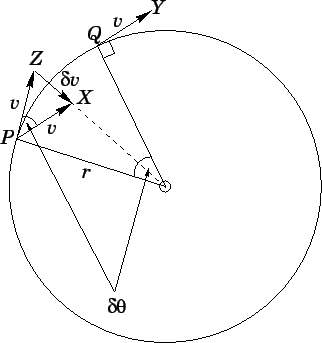Suppose that the object moves from point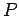to point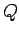between timesand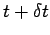, as shown in Fig. 58. Suppose, further, that the object rotates through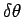radians in this time interval. The vector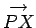, shown in the diagram, is identical to the vector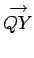. Moreover, the angle subtended between vectors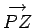andis simply. The vector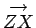represents the change in vector velocity,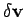, between timesand. It can be seen that this vector is directed towards the centre of the circle. From standard trigonometry, the length of vectoris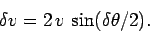(256)

However, for small angles, provided thatis measured in radians. Hence,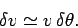(257)

It follows that(258)

where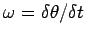is the angular velocity of the object, measured in radians per second. In summary, an object executing a circular orbit, radius, with uniform tangential velocity, and uniform angular velocity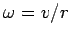, possesses an acceleration directed towards the centre of the circle--i.e., a centripetal acceleration--of magnitude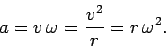(259)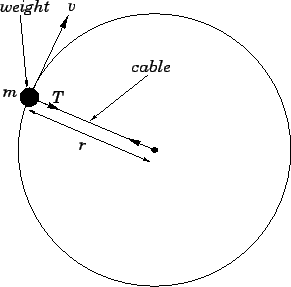Suppose that a weight, of mass, is attached to the end of a cable, of length, and whirled around such that the weight executes a horizontal circle, radius, with uniform tangential velocity. As we have just learned, the weight is subject to a centripetal acceleration of magnitude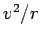. Hence, the weight experiences a centripetal force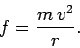(260)

What provides this force? Well, in the present example, the force is provided by the tension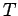in the cable. Hence,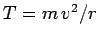.

Suppose that the cable is such that it snaps whenever the tension in it exceeds a certain critical value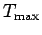. It follows that there is a maximum velocity with which the weight can be whirled around: namely,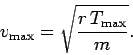(261)

Ifexceeds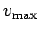then the cable will break. As soon as the cable snaps, the weight will cease to be subject to a centripetal force, so it will fly off--with velocity--along the straight-line which is tangential to the circular orbit it was previously executing.Next: The conical pendulum Up: Circular motion Previous: Uniform circular motion
Richard Fitzpatrick 2006-02-02